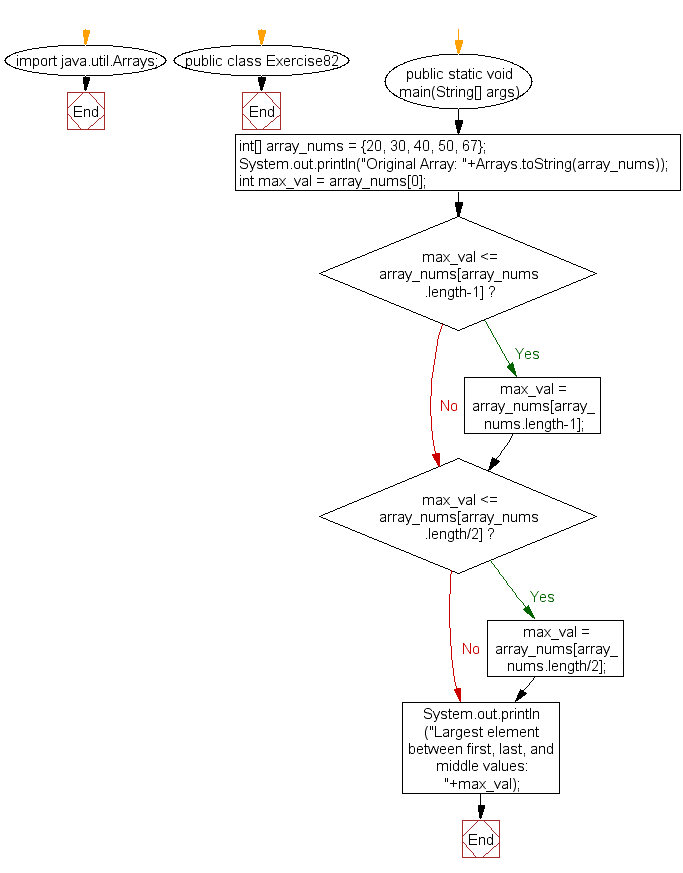﻿ Java: Largest value between first, last, and middle# Java Exercises: Find the largest element between first, last, and middle values from an array of integers

## Java Basic: Exercise-82 with Solution

Write a Java program to find the largest element between first, last, and middle values from an array of integers (even length).

Sample Solution:

Java Code:

``````import java.util.Arrays;
public class Exercise82 {
public static void main(String[] args)
{
int[] array_nums = {20, 30, 40, 50, 67};
System.out.println("Original Array: "+Arrays.toString(array_nums));
int max_val = array_nums;
if(max_val <= array_nums[array_nums.length-1])
max_val = array_nums[array_nums.length-1];
if(max_val <= array_nums[array_nums.length/2])
max_val = array_nums[array_nums.length/2];
System.out.println("Largest element between first, last, and middle values: "+max_val);
}
}
```
```

Sample Output:

```Original Array: [20, 30, 40, 50, 67]
Largest element between first, last, and middle values: 67
```

Flowchart:Java Code Editor:

What is the difficulty level of this exercise?

Test your Programming skills with w3resource's quiz.

﻿

## Java: Tips of the Day

countOccurrences

Counts the occurrences of a value in an array.

Use Arrays.stream().filter().count() to count total number of values that equals the specified value.

```public static long countOccurrences(int[] numbers, int value) {
return Arrays.stream(numbers)
.filter(number -> number == value)
.count();
}
```

Ref: https://bit.ly/3kCAgLb